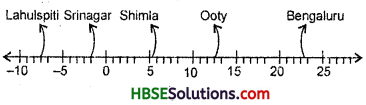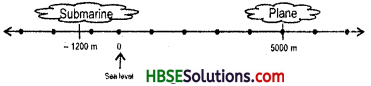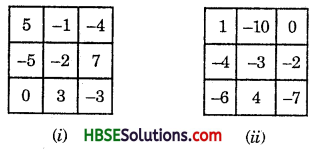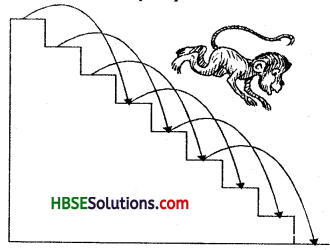# HBSE 7th Class Maths Solutions Chapter 1 Integers Ex 1.1

Haryana State Board HBSE 7th Class Maths Solutions Chapter 1 Integers Ex 1.1 Textbook Exercise Questions and Answers.

## Haryana Board 7th Class Maths Solutions Chapter 1 Integers Exercise 1.1

Question 1.
Following number line shows the temperature in degree celcius (°C) at different places on a particulars day.(a) Observe this number line and write the temperature of the places marked on it.
(b) What is the temperature difference between the hottest and the coldest places?
(c) What is the temperature difference between Lahulspiti and Srinagar ?
(d) Can we say temperature of Srinagar and Shimla taken together is less that the temperature at Shimla ? It is also less than the temperature at Srinagar.
Solution:
Temperature of hottest place (Bangalore) = 22°C
Temperature of coldest place (Lahulspiti)
= – 8°C
∴ The temperature difference between the hottest and coldest places
= 22°C – (- 8°C) = 22°C + 8° C = 30°C
Temperature of Lahulspiti = – 8°C
Temperature of Srinagar = – 2°C
The temperature difference between Lahulspiti and Srinagar = – 8°C – (- 2°C)
= – 8°C + 2°C = – 6°C
Temperature of Srinagar = – 2°C
Temperature of Shimla = 5°C Q.Question 2.
In a quiz, positive marks are given for correct answers and negative marks are given for incorrect answers. If Jack’s scores in five successive rounds were 25, – 5, – 10, 15, and 10. What was his total at the end ?
Solution:
Total score of Jack = 25 + (- 5) + (- 10) + 15 + 10
= 25-5-10 + 15 + 10 = 50 – 15 = 35

Question 3.
At Srinagar temperature was – 5°C on Monday and then it dropped by 2°C on Tuesday. What was the temeprature of Srinagar on Tuesday ? On Wednesday, it rose by 4°C. What was the temperature on this day ?
Solution:

 Days Temperature Monday -5°C Tuesday – 5°C – (2°C) = – 7°C Wednesday – 7°C + (4°C) = – 7°C + 4°C = – 3°C

Question 4.
A plane is flying at the height of 5000 m above the sea level. At a particular point it is exactly above a submarine floating 1200 m below the sea level. What is the vertical distance between them ?
Solution:Vertical distance between plane and submarine
= 5000 – (- 1200) = 5000 + 1200 = 6200 m.

Question 5.
Mohan deposits Rs. 2000 in his bank account and withdraws Rs. 1642 from it, the next day. If withdrawal of amount from the account is represented by a negative integer, then how will you represent the amount deposited ? Find the balance in Mohan’s account after the withdrawal.
Solution:
Balance in Mohan’s account = 2000 + (-1642) = 2000 – 1642 = Rs. 358

Question 6.
Rita goes 20 km towards east from a point A to the point B. From B, she moves 30 km towards west along the same road. If the distance towards east is represented by a positive integer then, how will you represent the distance travelled towards west ? By which integer will you represent her final position from A ?
Solution:
Final Integer =20 + (- 30) = 20-30 = -10.Question 7.
In a magic square each row, column and diagonal have the same sum. Check which of the following is a magic square.Solution:
(ii) Is a magic square
1 – 3 – 7 = -9
-6-3+0 = -9

1-4-6 =-9
-10 – 3 + 4 = -9
0 – 2 – 7 = -9

1 – 10 + 0 = -9
-4 – 3 – 2 =-9
-6 + 4 – 7 =-9

Question 8.
Verify a – (- b) = a + b for the following values of a and 6.
(i) a = 21, b = 18
(ii) a = 118, b = 125
(iii) a = 75, b = 84
(iv) a = 28, b = 11
Solution:
(i) 21 – (- 18) = 21 + 18 = 39
(ii) 118 – (- 125) = 118 + 125 = 243
(iii) 75 – (- 84) = 75 + 84 = 159
(iv) 28-(-11) = 28+ 11 = 39

Question 9.
Use the sign of >, < or = in the box to make the statement true.
(a) (-8) +(-4) □ (-8)-(-4)
(b) (-3) + 7 – (19) □ 15 – 8 + (- 9)
(c) 23-41 + 11 □ 23-41-11
(d) 39 + (-24) – (15) □ 36 + (-52)-(-36)
(e) – 231 + 79 + 51 □ -399 + 159 + 81
Solution:
(a) -8-4 < -8 + 4
(b) -3 + 7-19 < 15 – 8 – 9 ⇒ -12 < -4
-15 < -2
(c) 34 – 41 > 23 – 52
(d) 39 – 39 < 36 – 52 + 36 -7 > -29
0 < 20
(e) -231 + 130 > -399 + 240
-101 > -159Question 10.
A water tank has steps inside it. A Monkey is sitting on the topmost step (i.e., the first step). Water level is at the ninth step.
(i) He jumps 3 steps down and then jump 2 steps up. In how many jumps will he reach the water level?
(ii) After drinking water, he wants to go back. For this, he jumps 4 steps up and then jump back 2 steps down in every move. In how many jumps will he reach back the top step ?(iii) If the number of steps moved down is represented by a negative integers and the number of steps moved up by positive integers, represent his moves in Part (i) and (ii) by completing the following; (a) -3 + 2 + =-8
(b) 4-2 + = 8. In (a) the sum (- 8)
represents going down by eight steps. So, what will the sum 8 in (b) represent ?
Solution:
(i) Six Jumps, (ii) Three jumps.
(Hi) (a) — 3 + 2 — 3 + 2 — 3 + 2 — 3 + 2 – 3 + 2 – 3 = -8
⇒ -18 + 10 = 8
(b) 4-2 + 4- 2 + 4 = 8
⇒ 12 – 4 = 8
i.e., The sum (+ 8) represent going up by eight steps.